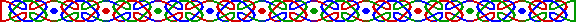「13」一數在西方世界中，一直視作為不祥之數。若是每月「13」日日碰上星期五，更被稱為「黑色星期五」(Black Friday) ，有的人在這天不會上班，有的人在這天不會上酒家吃飯，有的人在這天不會遠行、結婚、立約，大廈或酒店中找不到「13」樓等。外國人們對待「13」是敬而遠之，為什麼呢？「13」有什麼有趣事呢？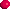把 13 的平方數 169 倒過來，即 961，這剛好是 31 的平方數。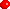13是最小的一個素數可以分拆成兩素數平方之和。

13 = 22 + 32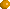13亦是最小的一個素數的數位總和 (Sum of Digits) 是一平方數 (Square Number)：

1 + 3 = 4 = 22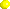13 減去其數位總和，我們得一平方數：

13 - (1+3) = 9 = 32

13 + (1*3) = 16 = 42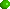13 是第一個反素數 (Emirp)，即把 13 反過來的 31 也是素數，詳見《反轉的素數》。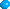而 1313 - 13 + 1 亦是一素數。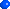把 1 至 13 的立方數 (Cubic Number) 連在一起：

2197172813311000729512343216125642781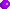現在已知的惟一一個存在於沙馬雲達基鏡數列 (Smarandache Mirror Sequence) 中素數正是第 13 個數：

1、 212、 32123、 4321234、 543212345、 65432123456、 7654321234567、 876543212345678、 98765432123456789、 109876543212345678910、 1110987654321234567891011、 12111098765432123456789101112、 131211109876543212345678910111213 1413121110987654321234567891011121314 ...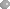13 亦是一威爾遜素數 (Wilson Prime) ，因 132 可整除 (13-1)!+1。而除了 13 以外，現在已知的威爾遜素數僅 5 和 563 兩員，詳見《小素民族》一文。其中一個超大素數 (Titanic Prime) 為 10999 + 13。最小的一個有十三位的素數為 1000000000039，其數位總和亦是 13。我們再看看：

132 = 169 和 312 = 961

(1+3)2 = 1+6+9

(3+1)2 = 9+6+1我們若把 13 和 31 放在一起： 1331 = 113132 = 169，但 169 亦非同小可，內藏不少平方數：

1+6+9 = 16 = 42

16+9 = 25 = 52

16*9 = 144 = 122

169 = (16+9) + (16*9)把 1 至 13 加在一起，我們得 91，而 91 是最小的一個數可以分別寫成兩數之立方差與兩數之立方和。

91 = 33 + 43

91 = 63 - 5313的倒數為 1/13 = 0.076923076923076923... ，我們以其中的 76923 來看看：

 76923 * 13 = 0999999 76923 * 26 = 1999998 76923 * 39 = 2999997 76923 * 52 = 3999996 76923 * 65 = 4999995 76923 * 78 = 5999994 76923 * 91 = 6999993 76923 * 104 = 7999992 76923 * 117 = 8999991 76923 * 130 = 9999990把不少於13的素數加起來， 2+3+5+7+11+13 = 41 ，這正是第 13 個素數。13 的英文 「Thirteen」亦是第一個以 「-teen」 結尾的數字。13 亦稱為「月數 (Moon Number)」，因為平均每一日月球移動 13度，且一年月球約繞地球 13 周。一很美麗的文字數式：

ELEVEN + TWO = TWELVE + ONE每一年也起碼有一次「黑色星期五」，有時一年中會多達三次之多， 1998 年便有三次 「黑色星期五」，而下一個有三次「黑色星期五」的年份是 2009 年。

Caldwell, Chris, Honaker, G. L. Jr., "13" From Prime Curios. http://primes.utm.edu/curios/page.php/13.html

Gupta, S. S. "Unlucky 13." From Number Recreations. http://www.shyamsundergupta.com/unlucky13.htm.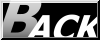Hosted by www.Geocities.ws